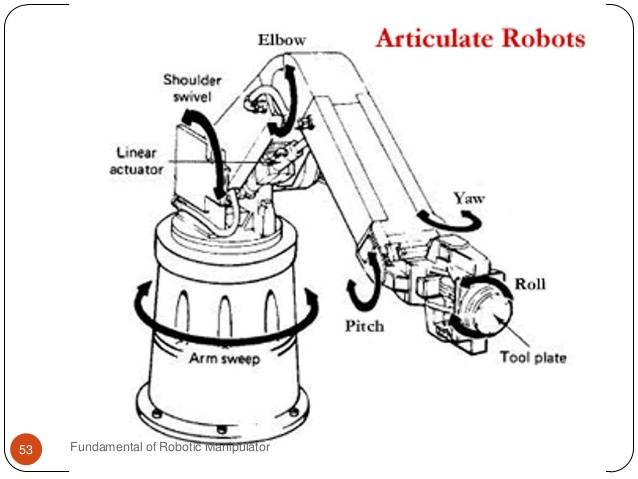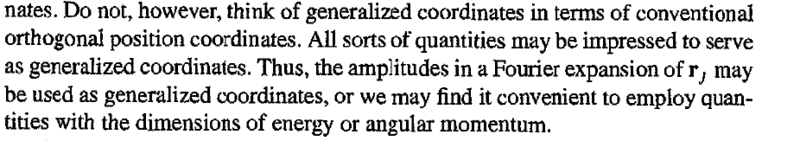# Amplitudes of Fourier expansion of a vector as the generalized coordinates

• I
RickRazor
When discussing about generalized coordinates, Goldstein says the following:

"All sorts of quantities may be impressed to serve as generalized coordinates. Thus, the amplitudes in a Fourier expansion of vector(rj) may be used as generalized coordinates, or we may find it convenient to employ quantities with the dimensions of energy or angular momentum."

I understand that generalized coordinates need not be orthogonal position vectors. But what does Fourier expansion of a vector even mean? A vector has 3 coordinates, now the amplitudes of Fourier expansion are infinite. How can they be used as generalized coordinates?

Gold Member
2022 Award
I've no clue. I'd need more context from the book (which I'd read with some care, because there's at least one strange mistake concerning anholonomous constraints, which has been discussed a while ago in the textbook forum).

Haborix
I suspect he means something like collective coordinates. This makes sense if what you call vector(rj) is the position of N particles indexed by your subscript, j.

•RickRazor
RickRazor
I've no clue. I'd need more context from the book (which I'd read with some care, because there's at least one strange mistake concerning anholonomous constraints, which has been discussed a while ago in the textbook forum).
He just starts talking about generalized coordinates and the transformation equations. He gives an example of the two angles theta1 and theta2 of a double pendulum and how the generalized coordinates need not be position vectors.

Gold Member
2022 Award
But that has nothing to do with Fourier transformations. Of course, a generalized coordinate can be anything describing the location of the system in its configuration space. Later you'll also learn about the Hamiltonian formulation, where you use phase space, i.e., generalized coordinates and their canonically conjugated momenta.

•RickRazor
I understand that generalized coordinates need not be orthogonal position vectors. But what does Fourier expansion of a vector even mean? A vector has 3 coordinates, now the amplitudes of Fourier expansion are infinite. How can they be used as generalized coordinates?
1. A vector can have any number of coordinates. 3 coordinates are just enough to pinpoint a position in a physical space, but in most cases you need more than that.
2. The Fourier series give you coordinates in a function space (usually of periodic functions with period 2π). Those coordinates approximate a function, not a point in space.

RickRazor
1. A vector can have any number of coordinates. 3 coordinates are just enough to pinpoint a position in a physical space, but in most cases you need more than that.
2. The Fourier series give you coordinates in a function space (usually of periodic functions with period 2π). Those coordinates approximate a function, not a point in space.
Can you explain what do you mean by requiring more than 3 coordinates for a vector in 3D space?

For 2) That's exactly what I'm not understanding. Goldstein said what he said.

Can you explain what do you mean by requiring more than 3 coordinates for a vector in a 3D space?
There are several examples, but my favorite example is from robotics.A robot manipulator must place its tool in the correct position (3 coordinates; x, y, z) with the correct orientation (3 coordinates; φ, ϑ, ψ) All in all - 6 coordinates.

Given the construction of the robot arm in the picture, it is fairly easy to calculate the position and orientation from the angles and lengths. The other way around is not trivial.

Hendrik Boom
The vector you get from Fourier coefficients is a vector in an infinite-dimensional space. This is definitely not the three-dimensional space we are all used to living in.

RickRazor
The vector you get from Fourier coefficients is a vector in an infinite-dimensional space. This is definitely not the three-dimensional space we are all used to living in.

But then, there was no context of infinite-dimensional spaceGold Member
2022 Award
As I already said, that's not enough context to make sense about this statement. I'd tend to think, it doesn't make sense here at all since for point mechanics you have a finite number of independent degrees of freedom in configuration space, and it doesn't make sense to use a Fourier transformation of some kind to relabel them.

This changes for the case of fields, where you have a continuous number of field-degrees of freedom, which you may either label in terms of ##\vec{x}## or of ##\vec{k}##, where
$$\phi(t,\vec{x})=\int_{\mathbb{R}^3} \frac{\mathrm{d}^3 \vec{k}}{(2 \pi)^3} \tilde{\phi}(t,\vec{k}) \exp(-\mathrm{i} \vec{k} \cdot \vec{x}).$$

•RickRazor
Hendrik Boom
No. But that's what you get with a Fourier analysis. One dimension for each frequency. It's possible to ignore all but a few more important coefficients, thereby making a more tractable finite-dimensional approximation, but that is an approximation.

RickRazor
As I already said, that's not enough context to make sense about this statement. I'd tend to think, it doesn't make sense here at all since for point mechanics you have a finite number of independent degrees of freedom in configuration space, and it doesn't make sense to use a Fourier transformation of some kind to relabel them.

This changes for the case of fields, where you have a continuous number of field-degrees of freedom, which you may either label in terms of ##\vec{x}## or of ##\vec{k}##, where
$$\phi(t,\vec{x})=\int_{\mathbb{R}^3} \frac{\mathrm{d}^3 \vec{k}}{(2 \pi)^3} \tilde{\phi}(t,\vec{k}) \exp(-\mathrm{i} \vec{k} \cdot \vec{x}).$$
Someone tells me that it has got something to do with Discrete Fourier Transform
$${\bf r}_j(t)~=~\sum_{k=1}^N e^{2\pi i jk/N }{\bf q}_j(t)$$

And that the amplitudes $${\bf q}_j(t)$$ play the role of generalized coordinates.